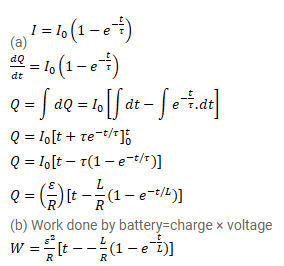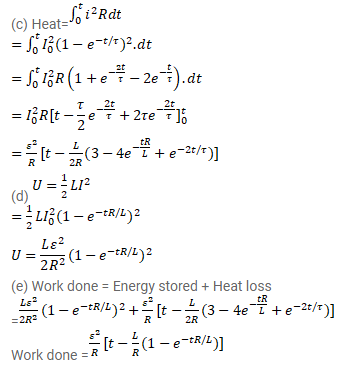# An LR circuit with emf ε is connected at t=0.

Question:

An LR circuit with emf $\varepsilon$ is connected at $\mathrm{t}=0$.

(a) Find the charge $Q$ which flows through the battery during 0 to t.

(b) Calculate the work done by the battery during this period.

(c) Find the heat developed during this period.

(d) Find the magnetic field energy stored in the circuit at time t.

(e) Verify that the results in the three parts above are consistent with energy conservation.

Solution: